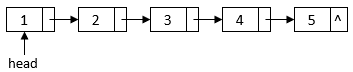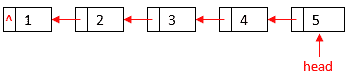### Get started with Spring 5 and Spring Boot 2, through the Learn Spring course:

>> CHECK OUT THE COURSE

## 1. Introduction

In this tutorial, we’ll implement two linked list reversal algorithms in Java.

## 2. Linked List Data Structure

A linked list is a linear data structure in which a pointer in each element determines the order. Each element of a linked list contains a data field to store the list data and a pointer field to point to the next element in the sequence. Also, we can use a head pointer to point to the start element of a linked list:After we reverse the linked list, the head will point to the last element of the original linked list, and the pointer of each element will point to the previous element of the original linked list:In Java, we have a LinkedList class to provide a doubly-linked list implementation of the List and Deque interfaces. However, we’ll use a general singly-linked list data structure in this tutorial.

``````public class ListNode {

private int data;
private ListNode next;

ListNode(int data) {
this.data = data;
this.next = null;
}

// standard getters and setters
}``````

The ListNode class has two fields:

• An integer value to represent the data of the element
• A pointer/reference to the next element

A linked list may contain multiple ListNode objects. For example, we can construct the above sample linked list with a loop:

``````ListNode constructLinkedList() {
ListNode tail = null;
for (int i = 1; i <= 5; i++) {
ListNode node = new ListNode(i);
} else {
tail.setNext(node);
}
tail = node;
}
}``````

## 3. Iterative Algorithm Implementation

Let’s implement the iterative algorithm in Java:

``````ListNode reverseList(ListNode head) {
ListNode previous = null;
while (current != null) {
ListNode nextElement = current.getNext();
current.setNext(previous);
previous = current;
current = nextElement;
}
return previous;
}``````

In this iterative algorithm, we use two ListNode variables, previous and current, to represent two adjacent elements in the linked list. For each iteration, we reverse these two elements and then shift to the next two elements.

In the end, the current pointer will be null, and the previous pointer will be the last element of the old linked list. Therefore, previous is also the new head pointer of the reversed linked list, and we return it from the method.

We can verify this iterative implementation with a simple unit test:

``````@Test
for (int i = 1; i <= 5; i++) {
assertNotNull(node);
assertEquals(i, node.getData());
node = node.getNext();
}

for (int i = 5; i >= 1; i--) {
assertNotNull(node);
assertEquals(i, node.getData());
node = node.getNext();
}
}``````

In this unit test, we first construct a sample linked list with five nodes. Also, we verify that each node in the linked list contains the correct data value. Then, we call the iterative function to reverse the linked list. Finally, we check the reversed linked list to make sure the data are reversed as expected.

## 4. Recursive Algorithm Implementation

Now, let’s implement the recursive algorithm in Java:

``````ListNode reverseListRecursive(ListNode head) {
return null;
}
}
return node;
}``````

In the reverseListRecursive function, we recursively visit each element in the linked list until we reach the last one. This last element will become the new head of the reversed linked list. Also, we append the visited element to the end of the partially reversed linked list.

Similarly, we can verify this recursive implementation with a simple unit test:

``````@Test
for (int i = 1; i <= 5; i++) {
assertNotNull(node);
assertEquals(i, node.getData());
node = node.getNext();
}

for (int i = 5; i >= 1; i--) {
assertNotNull(node);
assertEquals(i, node.getData());
node = node.getNext();
}
}``````

## 5. Conclusion

In this tutorial, we implemented two algorithms to reverse a linked list. As always, the source code for the article is available over on GitHub.

### Get started with Spring 5 and Spring Boot 2, through the Learn Spring course:

>> CHECK OUT THE COURSE